Definitions of Square Dance Calls and Concepts
Scatter Circulate [C3A]

Index -->  Plus  |  A1  |  A2  |  C1  |  C2  |  C3A  |  C3B  |  C4  |  NOL  |
Definitions (Text Only) -->  Plus  |  A1  |  A2  |  C1  |  C2  |  C3A  |  C3B  |  C4  |  NOL  |
 Find call:

 \$B8@8l(BFrom Parallel Two-Faced Lines and other applicable 2 x 4 formations (such as Facing Lines, Back-to-Back Lines, and some T-Bones). EN: 10
\$BJ?9T\$J(B Two-Faced Line \$B\$HB>\$NE,@Z\$J(B 2 x 4 formation (\$BNc\$(\$P8~\$+\$\$9g\$C\$?(B Line, \$BGXCf9g\$o\$;\$N(B Line, \$B\$=\$7\$F\$"\$k(B T-Bone) \$B\$+\$i!%(B JP: 10

Ends Split Circulate as Out-facing Centers Crossover Circulate as In-facing Centers Concentric (Box) Crossover Circulate. EN: 20
Ends \$B\$O(B Split Circulate \$B\$r(B, \$B30\$r8~\$\$\$?(B Centers \$B\$O(B Crossover Circulate \$B\$r(B, \$BCf\$r8~\$\$\$?(B Centers \$B\$O(B Concentric (Box) Crossover Circulate \$B\$r\$7\$^\$9!%(B JP: 20

Parallel Two-Faced Lines end in Parallel Waves. EN: 30
\$BJ?9T\$J(B Two-Faced Line \$B\$+\$i\$O(B, \$BJ?9T\$J(B Wave \$B\$G=*\$o\$j\$^\$9!%(B JP: 30

Parallel Two-Faced Lines \$B\$+\$i(B: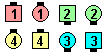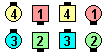Scatter Circulate\$B\$NA0(B \$B8e(B

T-Bone setup \$B\$+\$i(B: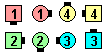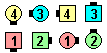Scatter Circulate\$B\$NA0(B \$B8e(B

\$BCm

• The In-Facing Center's part is usually danced as a Jaywalk, hence dancers should point at each other before moving. EN: 40
\$BCf\$r8~\$\$\$?(B centers \$B\$NF0\$-\$O(B, \$BIaDL(B Jaywalk \$B\$G9T\$o\$l\$F\$\$\$^\$9!%(B \$B\$7\$?\$,\$C\$F(B, \$BF0\$/A0\$K\$*8_\$\$\$K(B point \$B\$7\$J\$1\$l\$P\$J\$j\$^\$;\$s!%(B JP: 40
• From Parallel Lines, Scatter Circulate is equivalent to Stretched Box CirculateEN: 50
\$BJ?9T\$J(B Line \$B\$+\$i\$O(B, Scatter Circulate \$B\$O(B Stretched Box Circulate \$B\$HF1\$8\$G\$9!%(B JP: 50
• Funny Scatter Circulate  (from Parallel 3 & 1 Lines)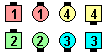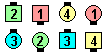Funny Scatter Circulate\$B\$NA0(B \$B8e(B

The above example is an instance of Funny where it is not those facing who do the call. The dancers who can move are those who can do the call in the normal manner without ending on the same spot as another dancer. EN: 60

Go First Class [C4]:
From Parallel Waves and other applicable formations. Leaders Switch the Wave as Center Trailers Concentric (Box) Crossover Circulate as End Trailers Split Circulate. Parallel Waves end in Parallel Two-Faced Lines. EN: 164
\$BJ?9T\$J(B Wave \$B\$^\$?\$OB>\$NE,@Z\$J(B formation \$B\$+\$i!%(B Leaders \$B\$,(B Switch the Wave \$B\$r\$9\$k4V\$K(B, Center Trailers \$B\$O(B Concentric (Box) Crossover Circulate \$B\$r(B, End Trailers \$B\$O(B Split Circulate \$B\$r\$7\$^\$9!%(B \$BJ?9T\$J(B Wave \$B\$+\$i\$O(B, \$BJ?9T\$J(B Two-Faced Line \$B\$G=*\$o\$j\$^\$9!%(B JP: 164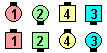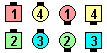Go First Class\$B\$NA0(B \$B8e(BCALLERLAB definition for Scatter CirculateChoreography for Scatter CirculateComments? Questions? Suggestions?